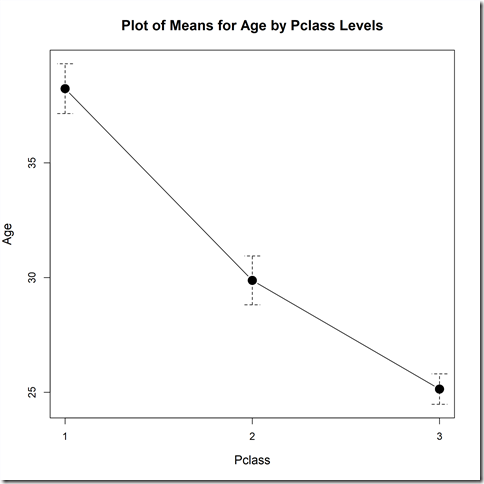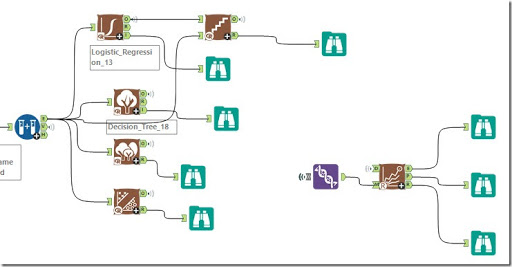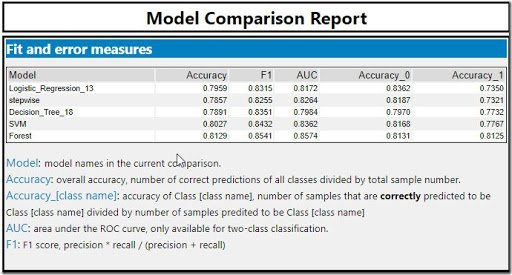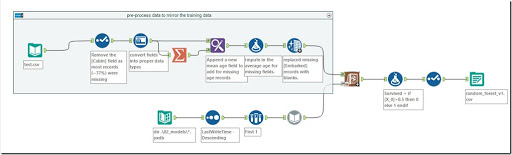# AT Data Science – Creating the Models

Last time we ran through the exploratory data analysis to# Pre-processing

The first step of creating models is the pre-processing of your data. Cleaning the data up to remove nulls, applying any scaling or normalising you need and potentially aggregating the raw data you are trying to predict.
For our data set we found there were a couple of fields with nulls which we need to tidy up. The first field we want to clean up is the [Age] field. When we look at the [Age] field we see there are 177 missing records. Additionally, we can apply some domain knowledge and ask the question, “Is there a difference in ages across the classes?” The theory being that the people travelling in the higher passenger classes are better off and possibly older.
When we look at the plot of means we can see that the average age in each class falls across the classes, using this insight each of the missing missing values was replaced by the mean for that class. The other field with missing values was the [Embarked] field. For this field we could replace the missing values with them mode or most common value, for the first iteration those missing values were just imputed with a blank field.# Modelling

The next step in the process is to create the models. For today, I’m just going to implement the standard models that Alteryx has built in (will investigate tuning the hyperparameters in a later blog).In Alteryx the building of initial models is super simple. When not looking at changing the hyperparameters all we are needing to consider is, what is the target variable and what are the input variables. For this analysis, I removed the [Name] and [Ticket] fields as they were identifier fields, rather than anything that would be generalisable. Additionally, while the Passenger ID is needed to match with the competition submissions but you dont want to include it in the modelling for the same reasons.

# Deciding on a Model

In our simple analysis the final step is to decide on what model you want to use. A really useful tool to use is the Model Comparison Macro. In the Alteryx gallery’s predictive district, there is a macro for quickly comparing different models to help decide on the best performing model.
In the picture below we see a table of performance characteristics that we can examine to decide on the best model. The main three metrics, Accuracy, F1 and AUC can be checked to decide on our preferred model. The Accuracy is a simple percentace of records predicted correctly. The F1 score is the harmonic mean between recall and precision, trying to find a balance between the two competing metrics. Finally, the AUC is the area under the ROC curve, the ROC curve or relative operating characteristics curve is, according to Wikipedia “is a graphical plot that illustrates the diagnostic ability of a binary classifier system as its discrimination threshold is varied.” basically the vertical access is the true positive rate (i.e. predict true and actually true), while the horizontal axis is the false positive rate (predicted true but actually false) and the further the line is in the top right corner the better. When higher in the top right corner the AUC returns the a higher value.when we look at the results for our initial models, we see the Forest model ends up coming out on top for all the key metrics of Accuracy at 0.8129, F1 at 0.8541 and AUC at 0.8574.

# Creating a SubmissionTo finish off our initial model building we need to apply our created model (the Random Forest) to the test set from the Kaggle competition. The first step is to apply the same pre-processing steps to the testing data that we applied to the training data. we also want to read the random forest model in (we saved it to a YXDB file). Finally, we apply the trained model to the processed data set using the scoring tool. This process gives us 2 columns with the probability of either a 1 or 0 for each record.
To create a the submission form we need to convert the probability (between 0 and 1) into a [Survived] column containing a 1 or 0. We also need to drop all columns that are not the Passenger ID or the Survived columns as detailed in the Kaggle submissions requirements.
When I submitted my created results the I was scored at a final 0.75119. this put me at 8652 on the scoreboard and puts a good point to keep building from.In a future post, I’m going to look at can we improve our random forest by tuning the hyperparameters, are any of the other models built into Alteryx any better for this problem (like a Neural network or Support Vector Machine) and what about the some of the other convolutional Neural networks available through Keras or Tensorflow